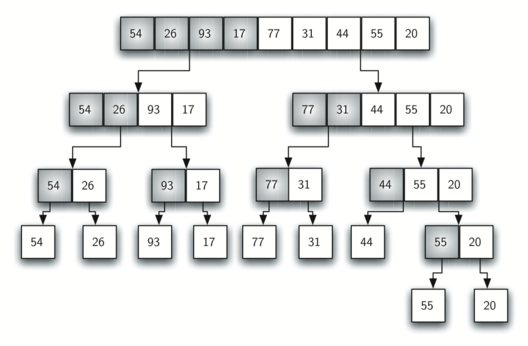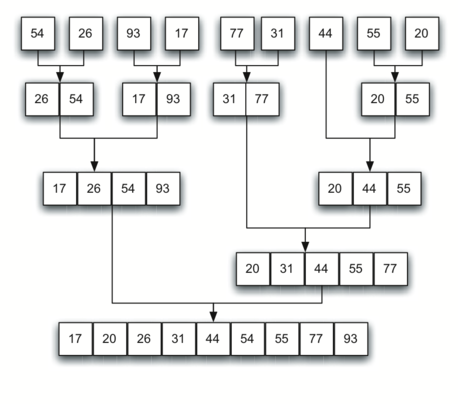# 6.11. The Merge Sort¶

We now turn our attention to using a divide and conquer strategy as a way to improve the performance of sorting algorithms. The first algorithm we will study is the merge sort. Merge sort is a recursive algorithm that continually splits a list in half. If the list is empty or has one item, it is sorted by definition (the base case). If the list has more than one item, we split the list and recursively invoke a merge sort on both halves. Once the two halves are sorted, the fundamental operation, called a merge, is performed. Merging is the process of taking two smaller sorted lists and combining them together into a single, sorted, new list. Figure 10 shows our familiar example list as it is being split by mergeSort. Figure 11 shows the simple lists, now sorted, as they are merged back together.Figure 10: Splitting the List in a Merge SortFigure 11: Lists as They Are Merged Together

The mergeSort function shown in ActiveCode 1 begins by asking the base case question. If the length of the list is less than or equal to one, then we already have a sorted list and no more processing is necessary. If, on the other hand, the length is greater than one, then we use the Python slice operation to extract the left and right halves. It is important to note that the list may not have an even number of items. That does not matter, as the lengths will differ by at most one.

Once the mergeSort function is invoked on the left half and the right half (lines 8–9), it is assumed they are sorted. The rest of the function (lines 11–31) is responsible for merging the two smaller sorted lists into a larger sorted list. Notice that the merge operation places the items back into the original list (alist) one at a time by repeatedly taking the smallest item from the sorted lists. Note that the statement lefthalf[i] <= righthalf[j] ensures that the algorithm is stable. A stable algorithm maintains the order of duplicate items in a list and is preferred in most cases.

The mergeSort function has been augmented with a print statement (line 2) to show the contents of the list being sorted at the start of each invocation. There is also a print statement (line 32) to show the merging process. The transcript shows the result of executing the function on our example list. Note that the list with 44, 55, and 20 will not divide evenly. The first split gives  and the second gives [55,20]. It is easy to see how the splitting process eventually yields a list that can be immediately merged with other sorted lists.

In order to analyze the mergeSort function, we need to consider the two distinct processes that make up its implementation. First, the list is split into halves. We already computed (in a binary search) that we can divide a list in half $$\log n$$ times where n is the length of the list. The second process is the merge. Each item in the list will eventually be processed and placed on the sorted list. So the merge operation which results in a list of size n requires n operations. The result of this analysis is that $$\log n$$ splits, each of which costs $$n$$ for a total of $$n\log n$$ operations. A merge sort is an $$O(n\log n)$$ algorithm.

Recall that the slicing operator is $$O(k)$$ where k is the size of the slice. In order to guarantee that mergeSort will be $$O(n\log n)$$ we will need to remove the slice operator. Again, this is possible if we simply pass the starting and ending indices along with the list when we make the recursive call. We leave this as an exercise.

It is important to notice that the mergeSort function requires extra space to hold the two halves as they are extracted with the slicing operations. This additional space can be a critical factor if the list is large and can make this sort problematic when working on large data sets.

Self Check